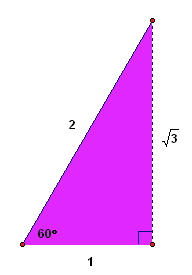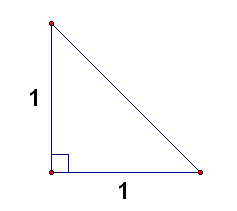﻿ Two Special Triangles
TWO SPECIAL TRIANGLES
• PRACTICE (online exercises and printable worksheets)

You should know the relationship between the lengths of the sides in two special triangles:

• $\,30^\circ{-}60^\circ{-}90^\circ\,$ triangles
• $\,45^\circ{-}45^\circ{-}90^\circ\,$ triangles

 $\,30^\circ\text{-}60^\circ\text{-}90^\circ\,$ TRIANGLE Lengths of Sides in a $\,30^\circ\text{-}60^\circ\text{-}90^\circ\,$ Triangle Let $\,s\,$ denote the length of the shortest side. Then, the hypotenuse has length $\,2s\,$. The side opposite the $\,60^\circ\,$ angle has length $\,\sqrt{3}s\,$. Conversely, if a triangle has sides of lengths $\,s\,$, $\,\sqrt{3}s\,$, and $\,2s\,$, then it is a $\,30^\circ\text{-}60^\circ\text{-}90^\circ\,$ triangle. Since $\,\sqrt{3}\approx 1.7\,$, it follows that the side opposite the $\,60^\circ\,$ angle is a little more than one and a half times the shortest side.
 $\,45^\circ\text{-}45^\circ\text{-}90^\circ\,$ TRIANGLE Lengths of Sides in a $\,45^\circ\text{-}45^\circ\text{-}90^\circ\,$ Triangle Let $\,s\,$ denote the length of the two shorter sides. Then, the hypotenuse has length $\,\sqrt{2}s\,$. Conversely, if a triangle has sides of lengths $\,s\,$, $\,s\,$, and $\,\sqrt{2}s\,$, then it is a $\,45^\circ\text{-}45^\circ\text{-}90^\circ\,$ triangle. Since $\,\sqrt{2}\approx 1.4\,$, it follows that the hypotenuse is a little less than one and a half times the shortest side.

It's easy to see that these are the correct relationships between the lengths of the sides, as follows:

$\,30^\circ\text{-}60^\circ\text{-}90^\circ\,$ Triangle Relationships
Start with an equiangular (hence equilateral) triangle, where each side has length $\,2\,$.
Notice that all the angles must be $\,\frac{180^\circ}{3} = 60^\circ\,$.
Drop a perpendicular, as shown below.
The purple and green triangles are $\,30^\circ\text{-}60^\circ\text{-}90^\circ\,$ triangles, where the length of the shortest side is $\,1\,$.
A quick application of the Pythagorean Theorem shows that the remaining side has length $\,\sqrt{3}\,$.
Then, scale all sides by $\,s\,$.$\,45^\circ\text{-}45^\circ\text{-}90^\circ\,$ Triangle Relationships
Create a triangle with two sides of length $\,1\,$ and a $\,90^\circ\,$ angle, as shown below.
Since angles opposite equal sides must be equal,
and since the two remaining angles must add to $\,90^\circ\,$,
they must each be $\,45^\circ\,$.
A quick application of the Pythagorean Theorem shows that the remaining side must equal $\,\sqrt{2}\,$.
Then, scale all sides by $\,s\,$.EXAMPLES:
Question:
In a $\,30^{\circ}\text{-}60^{\circ}\text{-}90^{\circ}\,$ triangle,
suppose that the length of the hypotenuse is $\,70\,$.
What is the length of the side opposite the $\,60^{\circ}\,$ angle?
Solution:
One strategy is to get the shortest side first, which is half the hypotenuse:
the shortest side is $\,\frac{70}2 = 35\,$.
Then, the side opposite the $\,60^{\circ}\,$ angle is $\,\sqrt{3}\,$ times as long, hence is $\,35\sqrt 3\,$.
Question:
Suppose that the lengths of the sides in a triangle are $\,13\,$, $\,13\sqrt{2}\,$, and $\,13\,$.
What type of triangle is this?
Solution:
This is a recognition question.
Notice that two sides are the same, and the other is $\,\sqrt{2}\,$ times as large.
This is a $\,45^{\circ}\text{-}45^{\circ}\text{-}90^{\circ}\,$ triangle.
This is the last exercise in Topics in Geometry.

(If you went through all the topics,
then congratulations are definitely in order!)

If you haven't already done so,
you might want to take a look at these:

A First Course in Algebra

Topics in Algebra II